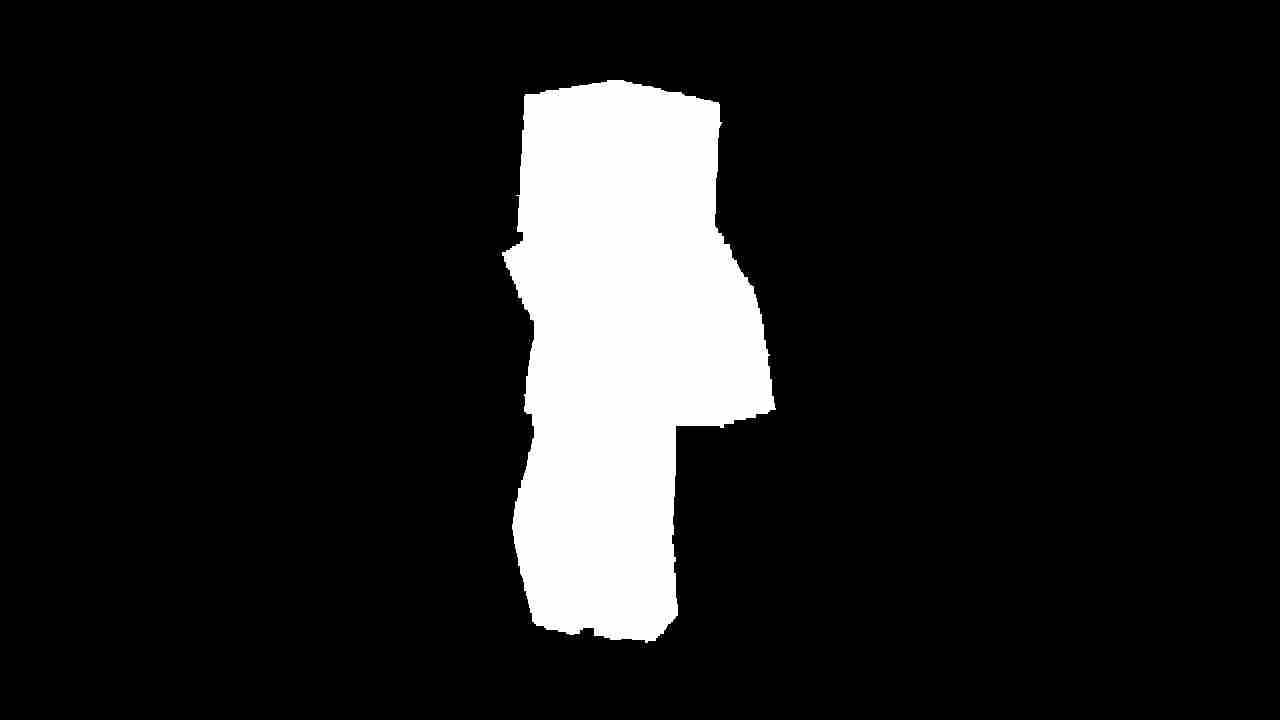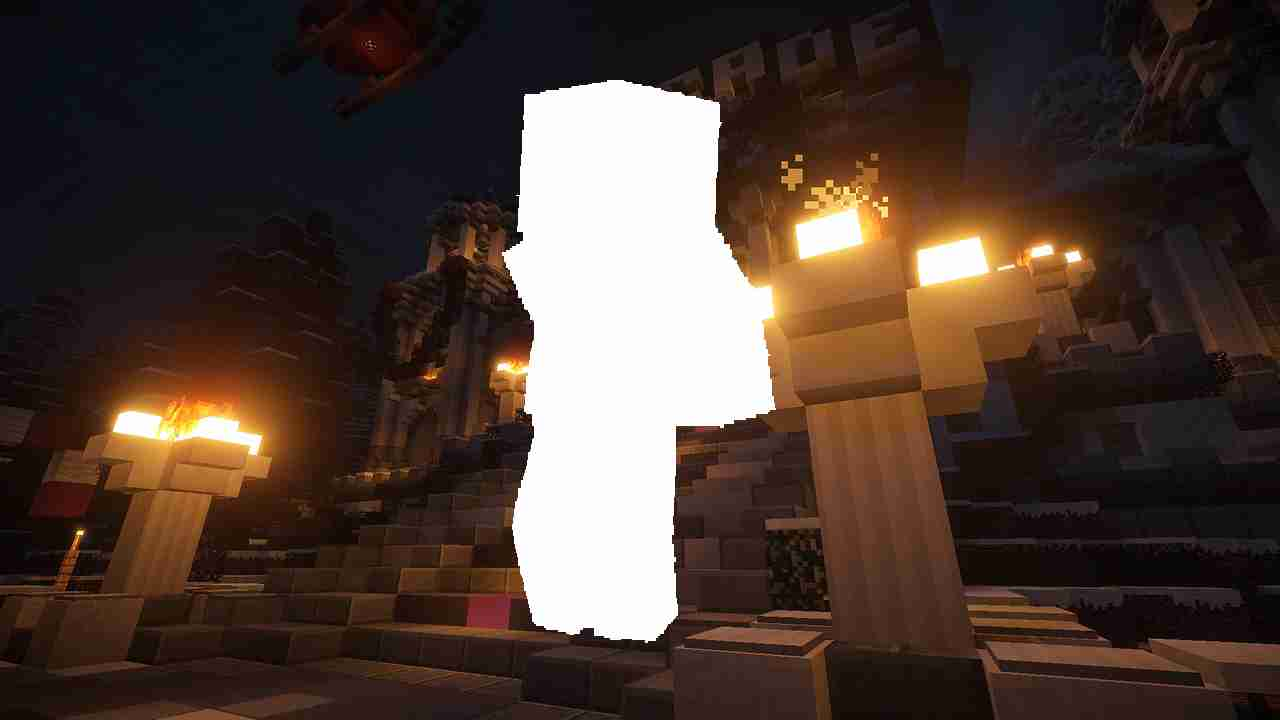# 计算机视觉项目系列*Python 3

*OpenCV 4.5.2

*绿屏视频。您可以使用此选项：

*替换背景的图像。您可以使用此选项：def create_mask_with_threshold(frame):    # split the the B, G and R channels    b, g, r = cv2.split(frame)    # create the threshold    _, mask = cv2.threshold(g, 245, 255, cv2.THRESH_BINARY_INV)    # De-noise the threshold to get a cleaner mask    mask = cv2.erode(mask, cv2.getStructuringElement(cv2.MORPH_RECT, (9, 9)))    return mask

# 热门人工智能文章：

def create_mask_with_in_range(frame):    mask = cv2.bitwise_not(cv2.inRange(frame, np.array([0, 200, 0]), np.array([0, 206, 0])))    mask = cv2.erode(mask, cv2.getStructuringElement(cv2.MORPH_RECT, (9, 9)))    return mask

• 创建当前帧的掩码版本• 否定面具
• 创建背景的遮罩版本• 合并两个蒙版图像def replace_background(frame, bg, mask):    # if the pixel on threshold is background then make it white    frame[mask == 0] = 255    # if the pixel on threshold is not background then make it black    bg[mask != 0] = 255    # combine both images into frame    return cv2.bitwise_and(bg, frame)

def replace_background_pixels(frame, bg, mask):    frame[mask == 0] = bg[mask == 0]    return frame

# 别忘了给我们你的ğŸ‘�！